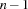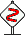## 4.2 General Purpose Search Engines

This section describes the search engines found in the module `Search`. All of these engines support recomputation, the possibility to stop their execution and various kinds of output.

Recomputation.

Scripts which create a large number of variables or propagators or scripts for which the search tree is very deep might use too much memory to be feasible. The search engines described in this section feature support for so-called recomputation. Recomputation reduces the space requirements for these scripts in that it trades space for time.

Search engines that do not use recomputation, create a copy of a computation space in each distribution step. This copy is needed such that the engine is able to follow more than one alternative of a choice.

If, for instance, a single solution search engine finds a solution after 200 distribution steps (i. e. the search tree has a depth of 201), 200 copies are created and stored by the engine.

Recomputation reduces the number of copies needed: Instead of creating a copy in each distribution step, only every-th distribution step a copy is created. A space for which no copy has been created can be recomputed from a copy located higher above in the search tree by recomputing some distribution steps. In the worst case,distribution steps have to be recomputed. The parameteris the so-called recomputation distance. A recomputation distance ofmeans that the space needed decreases by a factor ofand that the time needed increases by a factor of.

The following search engines take the recomputation distance as an argument (it is denoted by RcdI). A value of `2` for RcdI means that only each second distribution step a copy is created. The value `1` for RcdI means that in each distrbution step a copy is created, that is no recomputation is used. Values less than `1` mean that none but an initial copy is created: from this initial copy all other spaces are recomputed.

Recomputation can also reduce both space and time requirements. Searching a single solution of a script which features a good heuristic (i. e. there are only very few failures) creates copies which are not used. Recomputation avoids this, resulting in improvement with respect to both space and time.Recomputation requires that the distribution strategy used in the script be deterministic. Deterministic means that the created choices and their order are identical in repeated runs of the script. This is true for all strategies in the finite domain module, but for example not for strategies with randomized components.

Killing the Engine.

All engines described in this section return a nullary procedure, which is denoted by `+KillP`. Applying this procedure kills the search engine.

A search engine, which can be stopped and resumed is described in Section Section 4.3.

Different Types of Output.

Each of the engines is provided with three different types of output. The first kind returns a list of solutions as the engines in Section 4.1. The second kind returns a list of unary procedures. Applying one of these procedures merges a copy of the succeeded space and gives reference to its root variable variable by the actual argument of the procedure application. The third kind returns a list of succeeded spaces.

### 4.2.1 Single Solution Search

`one.depth`

`{Search.one.depth ``+ScriptP`` ``+RcdI``                    ``?KillP`` ``?Xs``}`

returns a singleton list containing the first solution of the script `+ScriptP` (a unary procedure) obtained by depth-first search. If no solution exists, `nil` is returned.

For instance, the procedure `Search.base.one` (see Section 4.1) can be defined as:

`fun {Search.base.one ScriptP}   {Search.one.depth ScriptP 1 _}end`

Suppose that `Script` is a script for which search does not terminate because it keeps on creating choices forever. It could look like the following:

`proc {Script X}   `···`    choice {Script X} [] {Script X} end end`

If `Search.one.depth` is applied to this particular script by

`Solutions={Search.one.depth Script 1 KillP}`

the search engine can be killed by applying `KillP` as follows:

`{KillP}`

Note that a script which keeps on computing forever even without search (i. e., because it contains an infinite recursion or loop) can not be killed.

`one.depthS`

`{Search.one.depthS ``+ScriptP`` ``+RcdI``                     ``?KillP`` ``?Spaces``}`

returns a singleton list containing the first succeeded space for the script `+ScriptP` (a unary procedure) obtained by depth-first search. If no solution exists, `nil` is returned.

`one.depthP`

`{Search.one.depthP ``+ScriptP`` ``+RcdI``                     ``?KillP`` ``?Ps``}`

Similar to `Search.one.depthS`, but returns a list of unary procedures as output.

`Search.one.depthP` can be defined using `Search.one.depthS` as follows:

`fun {Search.one.depthP Script RcdI ?KillP}   {Map thread            {Search.one.depthS Script RcdI ?KillP}        end         fun {\$ SuccSpace}           proc {\$ Root}              {Space.merge SuccSpace Root}           end         end}end`

`one.bound`

`{Search.one.bound ``+ScriptP`` ``+BoundI`` ``+RcdI`` ``?KillP`` ``?Xs``}`

`one.boundS`

`{Search.one.boundS ``+ScriptP`` ``+BoundI`` ``+RcdI`` ``?KillP`` ``?Spaces`

`one.boundP`

`{Search.one.boundP ``+ScriptP`` ``+BoundI`` ``+RcdI`` ``?KillP`` ``?Ps`

returns a singleton list containing the first solution of the script `+ScriptP` (a unary procedure) obtained by depth-first search, where the depth of the search tree explored is less than or equal to `+BoundI`.

If there is no solution in a depth less than or equal to `+BoundI`, but there might be solutions deeper in the tree, `cut` is returned. In case the entire search tree has a depth less than `+BoundI` and no solution exists, `nil` is returned.

Otherwise the output is a singleton list containing the solution (`Search.one.bound`), a succeeded space (`Search.one.boundS`), or a procedure (`Search.one.boundP`).

For instance

`{Search.one.bound proc {\$ X}                     choice fail [] fail end                   end                   1 1 _}`

returns the output `nil`, whereas

`{Search.one.bound proc {\$ X}                     choice                          choice fail [] fail end                      [] choice fail [] fail end                      end                   end                   1 1 _}`

returns the output `cut`.

`one.iter`

`{Search.one.iter ``+ScriptP`` ``+RcdI`` ``?KillP`` ``?Xs``}`

`one.iterS`

`{Search.one.iterS ``+ScriptP`` ``+RcdI`` ``?KillP`` ``?Spaces``}`

`one.iterP`

`{Search.one.iterP ``+ScriptP`` ``+RcdI`` ``?KillP`` ``?Ps``}`

returns a singleton list containing the first solution of the script `+ScriptP` (a unary procedure) obtained by iterative deepening depth-first search. If no solution exists, `nil` is returned.

Iterative deepening applies `Search.one.bound` to `+ScriptP` with depth-bounds 1, 2, 4, 8, ... until either a solution is found or `Search.one.bound` returns `nil`.

### 4.2.2 All Solution Search

`all`

`{Search.all ``+ScriptP`` ``+RcdI`` ``?KillP`` ``?Xs``}`

`allS`

`{Search.allS ``+ScriptP`` ``+RcdI`` ``?KillP`` ``?Spaces``}`

`allP`

`{Search.allP ``+ScriptP`` ``+RcdI`` ``?KillP`` ``?Ps``}`

returns the list of all solutions of the script `+ScriptP` (a unary procedure) obtained by depth-first search.

The output is a list of solutions (`Search.all`), a list of succeeded spaces (`Search.allS`), or a list of procedures (`Search.allP`).

### 4.2.3 Best Solution Search

`best.bab`

`{Search.best.bab ``+ScriptP`` ``+OrderP`` ``+RcdI`` ``?KillP`` ``?Xs``}`

`best.babS`

`{Search.best.babS ``+ScriptP`` ``+OrderP`` ``+RcdI`` ``?KillP`` ``?Spaces``}`

`best.babP`

`{Search.best.babP ``+ScriptP`` ``+OrderP`` ``+RcdI`` ``?KillP`` ``?Ps``}`

returns a singleton list containing the best solution with respect to the order `+OrderP` (a binary procedure) of the script `+ScriptP` (a unary procedure) obtained by branch and bound search. If no solution does exist, `nil` is returned.

The branch and bound strategy works as follows. When a solution is found, all the remaining alternatives are constrained to be better with respect to the order `+OrderP`. The binary procedure `+OrderP` is applied with its first argument being the previous solution, and its second argument the root variable of a space for one of the remaining alternatives.

`best.restart`

`{Search.best.restart ``+ScriptP`` ``+OrderP`` ``+RcdI`` ``?KillP`` ``?Xs``}`

`best.restartS`

`{Search.best.restartS ``+ScriptP`` ``+OrderP`` ``+RcdI`` ``?KillP`` ``?Spaces``}`

`best.restartP`

`{Search.best.restartP ``+ScriptP`` ``+OrderP`` ``+RcdI`` ``?KillP`` ``?Ps``}`

returns a singleton list containing the best solution with respect to the order `+OrderP` (a binary procedure) of the script `+ScriptP` (a unary procedure) obtained by branch and bound search. If no solution does exist, `nil` is returned.

The restart strategy works as follows. When a solution is found, search is restarted for `+ScriptP` with the additional constraint stating that the solution must be better with respect to the order `+OrderP`. The binary procedure `+OrderP` is applied with the previous solution as first argument, and the root variable of the script `+ScriptP` as its second argument.

Denys Duchier, Leif Kornstaedt, Martin Homik, Tobias Müller, Christian Schulte and Peter Van Roy
Version 1.4.0 (20080702)